# Linear Regression and Kernel Methods¶

Important: Please read the installation page for details about how to install the toolboxes. $\newcommand{\dotp}{\langle #1, #2 \rangle}$ $\newcommand{\enscond}{\lbrace #1, #2 \rbrace}$ $\newcommand{\pd}{ \frac{ \partial #1}{\partial #2} }$ $\newcommand{\umin}{\underset{#1}{\min}\;}$ $\newcommand{\umax}{\underset{#1}{\max}\;}$ $\newcommand{\umin}{\underset{#1}{\min}\;}$ $\newcommand{\uargmin}{\underset{#1}{argmin}\;}$ $\newcommand{\norm}{\|#1\|}$ $\newcommand{\abs}{\left|#1\right|}$ $\newcommand{\choice}{ \left\{ \begin{array}{l} #1 \end{array} \right. }$ $\newcommand{\pa}{\left(#1\right)}$ $\newcommand{\diag}{{diag}\left( #1 \right)}$ $\newcommand{\qandq}{\quad\text{and}\quad}$ $\newcommand{\qwhereq}{\quad\text{where}\quad}$ $\newcommand{\qifq}{ \quad \text{if} \quad }$ $\newcommand{\qarrq}{ \quad \Longrightarrow \quad }$ $\newcommand{\ZZ}{\mathbb{Z}}$ $\newcommand{\CC}{\mathbb{C}}$ $\newcommand{\RR}{\mathbb{R}}$ $\newcommand{\EE}{\mathbb{E}}$ $\newcommand{\Zz}{\mathcal{Z}}$ $\newcommand{\Ww}{\mathcal{W}}$ $\newcommand{\Vv}{\mathcal{V}}$ $\newcommand{\Nn}{\mathcal{N}}$ $\newcommand{\NN}{\mathcal{N}}$ $\newcommand{\Hh}{\mathcal{H}}$ $\newcommand{\Bb}{\mathcal{B}}$ $\newcommand{\Ee}{\mathcal{E}}$ $\newcommand{\Cc}{\mathcal{C}}$ $\newcommand{\Gg}{\mathcal{G}}$ $\newcommand{\Ss}{\mathcal{S}}$ $\newcommand{\Pp}{\mathcal{P}}$ $\newcommand{\Ff}{\mathcal{F}}$ $\newcommand{\Xx}{\mathcal{X}}$ $\newcommand{\Mm}{\mathcal{M}}$ $\newcommand{\Ii}{\mathcal{I}}$ $\newcommand{\Dd}{\mathcal{D}}$ $\newcommand{\Ll}{\mathcal{L}}$ $\newcommand{\Tt}{\mathcal{T}}$ $\newcommand{\si}{\sigma}$ $\newcommand{\al}{\alpha}$ $\newcommand{\la}{\lambda}$ $\newcommand{\ga}{\gamma}$ $\newcommand{\Ga}{\Gamma}$ $\newcommand{\La}{\Lambda}$ $\newcommand{\si}{\sigma}$ $\newcommand{\Si}{\Sigma}$ $\newcommand{\be}{\beta}$ $\newcommand{\de}{\delta}$ $\newcommand{\De}{\Delta}$ $\newcommand{\phi}{\varphi}$ $\newcommand{\th}{\theta}$ $\newcommand{\om}{\omega}$ $\newcommand{\Om}{\Omega}$ $\newcommand{\eqdef}{\equiv}$

This tour studies linear regression method, and its non-linear variant using kernlization.

We recommend that after doing this Numerical Tours, you apply it to your own data, for instance using a dataset from LibSVM.

Disclaimer: these machine learning tours are intended to be overly-simplistic implementations and applications of baseline machine learning methods. For more advanced uses and implementations, we recommend to use a state-of-the-art library, the most well known being Scikit-Learn

In :
using PyPlot
using NtToolBox

WARNING: Method definition ndgrid(AbstractArray{T<:Any, 1}) in module NtToolBox at /Users/gpeyre/.julia/v0.5/NtToolBox/src/ndgrid.jl:3 overwritten at /Users/gpeyre/.julia/v0.5/NtToolBox/src/ndgrid.jl:3.
WARNING: Method definition ndgrid(AbstractArray{#T<:Any, 1}, AbstractArray{#T<:Any, 1}) in module NtToolBox at /Users/gpeyre/.julia/v0.5/NtToolBox/src/ndgrid.jl:6 overwritten at /Users/gpeyre/.julia/v0.5/NtToolBox/src/ndgrid.jl:6.
WARNING: Method definition ndgrid_fill(Any, Any, Any, Any) in module NtToolBox at /Users/gpeyre/.julia/v0.5/NtToolBox/src/ndgrid.jl:13 overwritten at /Users/gpeyre/.julia/v0.5/NtToolBox/src/ndgrid.jl:13.
WARNING: Method definition ndgrid(AbstractArray{#T<:Any, 1}...) in module NtToolBox at /Users/gpeyre/.julia/v0.5/NtToolBox/src/ndgrid.jl:19 overwritten at /Users/gpeyre/.julia/v0.5/NtToolBox/src/ndgrid.jl:19.
WARNING: Method definition meshgrid(AbstractArray{T<:Any, 1}) in module NtToolBox at /Users/gpeyre/.julia/v0.5/NtToolBox/src/ndgrid.jl:33 overwritten at /Users/gpeyre/.julia/v0.5/NtToolBox/src/ndgrid.jl:33.
WARNING: Method definition meshgrid(AbstractArray{#T<:Any, 1}, AbstractArray{#T<:Any, 1}) in module NtToolBox at /Users/gpeyre/.julia/v0.5/NtToolBox/src/ndgrid.jl:36 overwritten at /Users/gpeyre/.julia/v0.5/NtToolBox/src/ndgrid.jl:36.
WARNING: Method definition meshgrid(AbstractArray{#T<:Any, 1}, AbstractArray{#T<:Any, 1}, AbstractArray{#T<:Any, 1}) in module NtToolBox at /Users/gpeyre/.julia/v0.5/NtToolBox/src/ndgrid.jl:44 overwritten at /Users/gpeyre/.julia/v0.5/NtToolBox/src/ndgrid.jl:44.


We test the method on the Boston house prices dataset, consisting in $n=506$ samples with features $x_i \in \RR^p$ in dimension $p=13$. The goal is to predict the price value $y_i \in \RR$.

Helpers.

In :
# SetAR = @(ar)set(gca, 'PlotBoxAspectRatio', [1 ar 1], 'FontSize', 10);
Xm = X -> X - repeat(mean(X,1), outer=(size(X,1), 1))
Cov = X -> Xm(X)'*Xm(X);


In :
A = readdlm("NtToolBox/src/data/boston_house_prices.csv", ',');


Randomly permute it.

In :
A = A[randperm(size(A,1)),:];


Separate the features $X$ from the data $y$ to predict information.

In :
X = A[:,1:end-1]
y = A[:,end];


$n$ is the number of samples, $p$ is the dimensionality of the features,

In :
n,p = size(X);


## Dimenionality Reduction and PCA¶

In order to display in 2-D or 3-D the data, dimensionality is needed. The simplest method is the principal component analysis, which perform an orthogonal linear projection on the principal axsis (eigenvector) of the covariance matrix.

Display the covariance matrix.

In :
imshow(Cov(X), extent=[0, 1, 0, 1], cmap = get_cmap("jet"));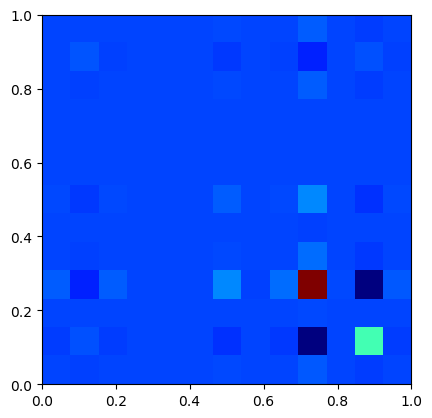Compute PCA ortho-basis and the feature in the PCA basis.

In :
U,D,V = svd(Xm(X),thin=true)
Z = Xm(X) * V;


Plot sqrt of the eigenvalues.

In :
figure(figsize=(5,3))
plot(D, ".-", linewidth= 2, markersize= 20); axis("tight");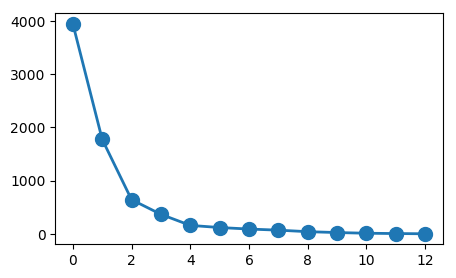Display the points cloud of feature vectors in 3-D PCA space.

In :
figure(figsize=(6,4))
plot_multiclasses(X,ones(n,1),disp_dim=3, ms=5);

UndefVarError: plot_multiclasses not defined

in include_string(::String, ::String) at /Applications/Julia-0.5.app/Contents/Resources/julia/lib/julia/sys.dylib:?

1D plot of the function to regress along the main eigenvector axes.

In :
col = ["blue" "green" "red" "cyan" "magenta" "yellow" "black"]
for i=1:min(p,3)
subplot(3,1,i)
plot(Z[:,i], y, ".", c=col[i], ms=10)
axis("tight")
end
tight_layout()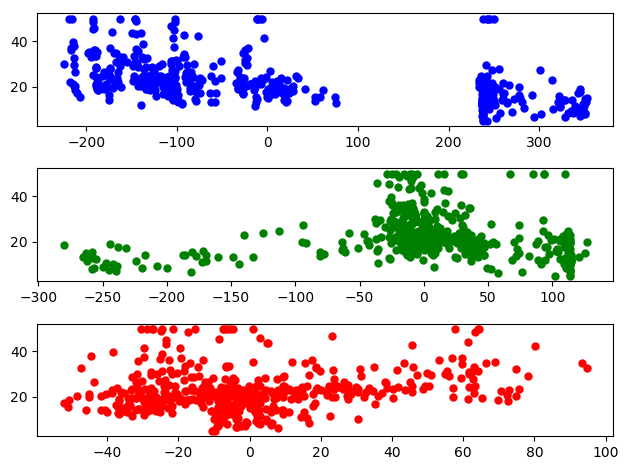## Linear Regression¶

We look for a linear relationship $y_i = \dotp{w}{x_i}$ written in matrix format $y= X w$ where the rows of $X \in \RR^{n \times p}$ stores the features $x_i \in \RR^p$.

Since here $n > p$, this is an over-determined system, which can solved in the least square sense $$\umin{ w } \norm{Xw-y}^2$$ whose solution is given using the Moore-Penrose pseudo-inverse $$w = (X^\top X)^{-1} X^\top y$$

Split into training and testing.

In :
n0 = Int(round(.5*n)); n1 = n-n0
X0 = X[1:n0,:];     y0 = y[1:n0]
X1 = X[n0+1:end,:]; y1 = y[n0+1:end];


Least square solution.

In :
w = (X0'*X0) \ (X0'*y0);


Mean-square error on testing set.

In :
E = sqrt( sum( (X1*w-y1).^2 ) / n1 );


Regularization is obtained by introducing a penalty. It is often called ridge regression, and is defined as $$\umin{ w } \norm{Xw-y}^2 + \lambda \norm{w}^2$$ where $\lambda>0$ is the regularization parameter.

The solution is given using the following equivalent formula $$w = (X^\top X + \lambda \text{Id}_p )^{-1} X^\top y,$$ $$w = X^\top ( XX^\top + \lambda \text{Id}_n)^{-1} y,$$ When $p<n$ (which is the case here), the first formula should be prefered.

In contrast, when the dimensionality $p$ of the feature is very large and there is little data, the second is faster. Furthermore, this second expression is generalizable to Kernel Hilbert space setting, corresponding possibly to $p=+\infty$ for some kernels.

In :
lambda = .1;
w = (X0'*X0+lambda*eye(p)) \ (X0'*y0);
w1 = X0'*( (X0*X0'+lambda*eye(n0)) \ y0 );
@printf "Error (should be 0): %.4f\n" norm(w-w1)/norm(w)

Error (should be 0): 0.0000


Exercise 1

Display the evolution of the error $E$ as a function of $\lambda$. Display error evolution.

In :
include("NtSolutions/ml_2_regression/exo1.jl");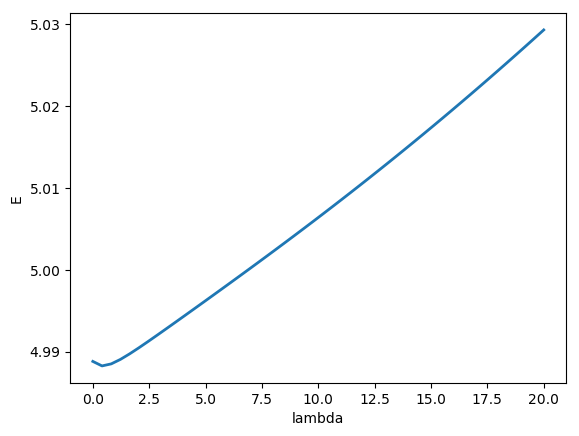In :
# Insert your code here.


Exercise 2

Display the regularization path, i.e. the evolution of $w$ as a function of $\lambda$.

In :
include("NtSolutions/ml_2_regression/exo2.jl");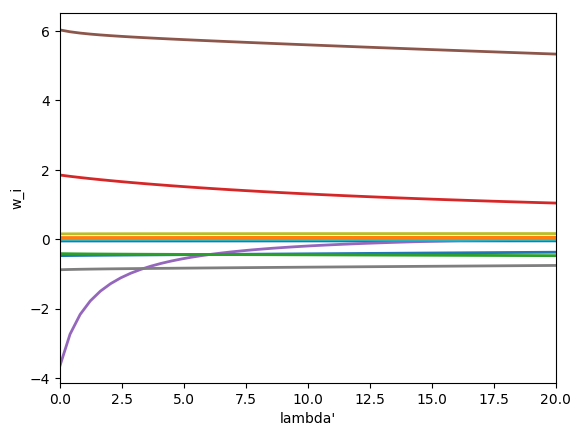In :
# Insert your code here.


Exercise 3

Perform feature selection using $\ell^1$ regularization (aka the Lasso), $$\umin{ w } \frac{1}{2}\norm{Xw-y}^2 + \lambda \norm{w}_1.$$

In :
include("NtSolutions/ml_2_regression/exo3.jl");

In :
# Insert your code here.


## Kernelized Ridge Regression¶

In order to perform non-linear and non-parametric regression, it is possible to use kernelization. It is non-parametric in the sense that the number of parameter grows with the number $n$ of samples (while for the initial linear method, the number of parameter is $p$). This allows in particular to generate estimator of arbitrary complexity.

Given a kernel $\kappa(x,z) \in \RR$ defined for $(x,z) \in \RR^p \times \RR^p$, the kernelized method replace the linear approximation functional $f(x) = \dotp{x}{w}$ by a sum of kernel centered on the samples $$f_h(x) = \sum_{i=1}^n h_i k(x_i,x)$$ where $h \in \RR^n$ is the unknown vector of weight to find.

When using the linear kernel $\kappa(x,y)=\dotp{x}{y}$, one retrieves the previously studied linear method.

Generate synthetic data in 2D. Add noise to a deterministic map.

In :
B = 3
n = 500; p = 2
# X = 2*B*rand(n,2)-B
# rho = .5; # noise level
# y = peaks(X[:,1], X[:,2]) + randn(n,1)*rho;


Display as scattered plot.

In :
scatter(X[:,1], X[:,2], ones(n,1)*20, y, cmap = get_cmap("jet"))
axis([-B B -B B]'); box("on");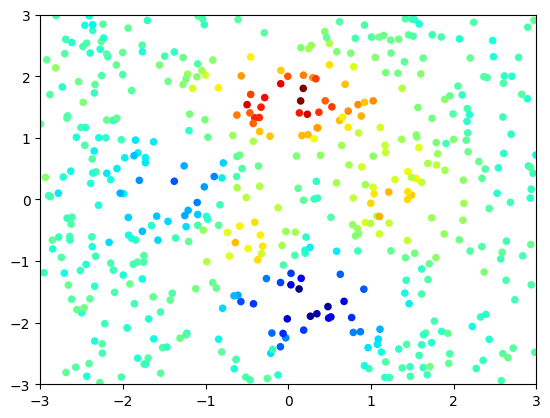Macro to compute pairwise squared Euclidean distance matrix.

In :
distmat = (X,Z) -> broadcast(+,sum(X'.*X',1)',sum(Z'.*Z',1))-2*(X*Z');


The gaussian kernel is the most well known and used kernel $$\kappa(x,y) \eqdef e^{-\frac{\norm{x-y}^2}{2\sigma^2}} .$$ The bandwidth parameter $\si>0$ is crucial and controls the locality of the model. It is typically tuned through cross validation.

In :
sigma = .3
kappa = (X,Z) -> exp( -distmat(X,Z)/(2*sigma^2) );


Once avaluated on grid points, the kernel define a matrix $$K = (\kappa(x_i,x_j))_{i,j=1}^n \in \RR^{n \times n}.$$

In :
K = kappa(X,X);


The weights $h \in \RR^n$ are solutions of $$\umin{h} \norm{Kh-y}^2 + \la \dotp{Kh}{h}$$ and hence can be computed by solving a linear system $$h = (K+\la \text{Id}_n)^{-1} y$$

In :
lambda = 0.01
h = (K+lambda*eye(n))\y;


Regressor.

In :
Y = x-> kappa(x,X)*h;


Evaluation on a 2D grid.

In :
q = 101;
t = linspace(-B,B,q);
v,u = meshgrid(t,t);
Xn = [u[:] v[:]];


Display as an image.

In :
yn = reshape(Y(Xn),q,q)
imshow(yn, extent=[0,1, 0,1], cmap = get_cmap("jet"))
axis("image"); axis("off");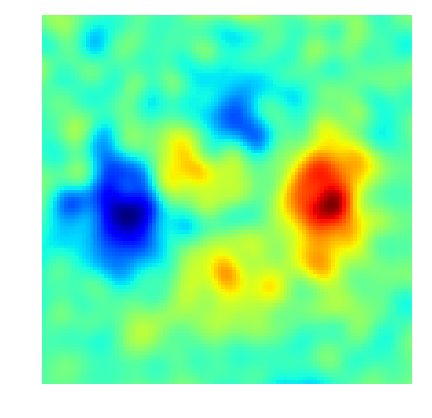Exercise 4

Display the evolution of the regression as a function of $\sigma$.

In :
include("NtSolutions/ml_2_regression/exo4.jl");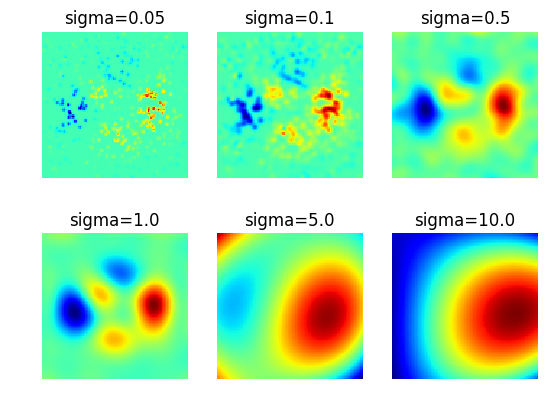In :
# Insert your code here.


Exercise 5

Apply the kernelize regression to a real life dataset. Study the influence of $\la$ and $\si$.

In :
include("NtSolutions/ml_2_regression/exo5.jl");

In :
# Insert your code here.